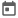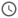# Interface in SV##### 26 Dec 2022##### 5 mins

In Verilog, we are used to defining ports while writing the modules. These ports act as the connection between different modules or between the design and the test bench. Defining ports and maintaining connections becomes a lot more difficult when the number of signals inside a port is very high. In System Verilog, interfaces were introduced. An interface can be thought of as a bundle of all the nets present in a port. So now, instead of individually defining signals in port, an interface can be used. Let's dig deep into the interface and how it is helpful in this article. In this article, we'll take a closer look at interfaces and how they can help in digital design projects, especially in test benches.

### Introduction

An interface in SystemVerilog is an entity that encapsulates all the signals into a single block. This enables us to pass all the signals inside an interface with ease within the testbench. Interfaces are static, which means that any change made in one instance is reflected in all other instances.

Interfaces are declared using the `interface` keyword and the declaration ends with the `endinterface` keyword. It is recommended to use the `logic` data type for all the nets in an interface. As we know, logic can be used both as a wire and a reg, so using logic makes the defined nets more flexible.

Logic is recommended because inside interface signals can act as both as input and output. In later stages we will learn about the modports and clocking blocks which will help in understanding this concept.

#### Declaration of interface

Inside an interface, signals can either be declared as arguments or as members of the interface. Both methods are correct and used depending on the specific use cases. However, it is more common to declare signals as members of an interface, especially when the interface is intended to be used at a lower level in the design hierarchy. This is because at the time of instantiation, the signals would not be available if they were declared as arguments.

Some top-level signals such as clk and rst are generally declared as arguments, as these signals are typically shared by multiple components and are often passed to the interface when it is instantiated. This can make it easier to connect the interface to the rest of the design and to manage the signal connections between the different components.

#### Syntax with signals as arguments

``````interface <interface_name> (<signals>)
…
endinterface``````

#### Syntax with signals as members

``````interface <interface_name> ([optional arguments])
<signals>
…
endinterface``````

### Parameterized interface

Just like modules, interfaces in SystemVerilog can also be parameterized. This is beneficial in scenarios where you want to reuse the ports but need some of the ports to have different widths. For example, in AXI, the data bus width can change depending on the design. By using a parameterized interface, you can define a single interface and adjust the width as needed for the specific design. Like a non-parameterized interface, a parameterized interface can be declared in two ways, like the examples shown above.

#### Syntax

``````interface <interface_name> #(parameters) ([optional arguments])
<signals>
…
endinterface``````

### Need for an interface

Interfaces are generally very much helpful in writing testbench. This is because most of the time designs are written in Verilog which won’t support the interface. In the test bench, we know there are lots of different components and the signal from the DUT needs to be connected to these components. The interface provides a simple encapsulation which can be reused in all the components and can be used to connect to DUT.

Interfaces are static and thus, can be easily called inside a module. Also, due to its static nature, the interface is an appropriate location to write assertions or logic which require raw signals and not the sampled signals.

Interfaces also provide various advanced features which we will learn about in future articles. To give a basic idea, inside an interface, different ports can be declared and each port can have different directions for the signals. Interfaces also help in synchronizing different signals with respect to a clock, which helps to avoid race conditions while driving or monitoring signals through the testbench.

### Example scenario 1

Let’s see how to create an interface for a design where there are 2 input ports and 1 output port. Also, for this scenario let's consider the design is written in SV and thus interface is used in the design as well.

In the interface shown in the example, we have used logic as the data type for the signals. To run any test on dut, we need to provide inputs to the dut. These will be driven by the test bench and thus with respect to the test bench these signals will be output and the output from dut will be the input. `logic` type helps in this as it can act both as reg and wire.

#### Code

``````//  demo interface with 2 inputs and 1 output.
interface demo_intf;
logic in1;
logic in2;
logic out1;

endinterface //demo_intf

//  example rtl which outputs xor of 2 inputs
module ex_dut(demo_intf intf);
endmodule

//  testbench where interface and dut is instantiated and connected.
//  As tb is small and only one heirarchy is present thus both way of
//  declaring interface as discussed is okay to use.
module tb_top();
demo_intf intf();
ex_dut dut(intf);

initial begin
\$monitor("in1 = %b, in2 = %b, out1 = %b",intf.in1, intf.in2, intf.out1);

intf.in1 <= 1'b1;
intf.in2 <= 1'b0;

#20;
intf.in1 <= 1'b1;
intf.in2 <= 1'b1;

#20;
intf.in1 <= 1'b0;
intf.in2 <= 1'b1;
end
endmodule``````
##### Output
``````# in1 = 1, in2 = 0, out1 = 1
# in1 = 1, in2 = 1, out1 = 0
# in1 = 0, in2 = 1, out1 = 1``````

### Example scenario 2

Let’s see how to create an interface for a design where there are 2 input signals, `in1` and `in2`, and 1 output signal namely `out1`. In this scenario, let's consider the design is written in Verilog and the width of signals can change in change. So, we will use a parametrized interface in this case.

#### Code

Design written in verilog, thus cannot use the interface.

``````module demo_dut#(WIDTH) (
input [WIDTH-1:0]   in1,
in2,
output [WIDTH-1:0]  out1
);

assign out1 = in1 ^ in2;
endmodule``````

Test bench and the interaface is written below.

``````//  demo interface with 2 inputs and 1 output.
`include "intf_dut_demo.v"
interface demo_intf #(parameter WIDTH);
logic [WIDTH-1:0] in1;
logic [WIDTH-1:0] in2;
logic [WIDTH-1:0] out1;
endinterface //demo_intf

//  testbench where interface and dut is instantiated and connected.
//  As tb is small and only one heirarchy is present thus both way of
//  declaring interface as discussed is okay to use.
module tb_top();
parameter WIDTH = 4;
demo_intf#(WIDTH) intf();
//  here instead of passing the interface directly we are passing
//  individual signals to the dut
demo_dut#(WIDTH) dut(
.in1(intf.in1),
.in2(intf.in2),
.out1(intf.out1)
);

initial begin
\$monitor("in1 = %b, in2 = %b, out1 = %b",intf.in1, intf.in2, intf.out1);

intf.in1 <= 4'b1100;
intf.in2 <= 4'b0011;

#20;
intf.in1 <= 4'b1;
intf.in2 <= 4'b1;

#20;
intf.in1 <= 4'b1010;
intf.in2 <= 4'b0101;
end
endmodule``````
##### Output
``````# in1 = 1100, in2 = 0011, out1 = 1111
# in1 = 0001, in2 = 0001, out1 = 0000
# in1 = 1010, in2 = 0101, out1 = 1111``````

Although an interface might not seem necessary in this small testbench example, it can be very useful when working with larger testbenches that have many components and lots of signals. By encapsulating all the signals into a single interface, you can easily pass them between different components and keep track of your signal connections.